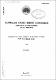## Analysis of flow stability in boiling systems with the TOSCLE code1971-05
##### Publisher
Australian Atomic Energy Commission
##### Abstract
Two-phase-flow stability can be analysed with the TOSCLE code. TOSCLE uses equations derived by linearising and taking Laplace transformations of the mass, energy and momentum conservation equations and solving the resultant one-space-dimension equations analytically along a straight, uniform cross-section pipe with uniform heat addition or removal per unit length. Separate solutions are obtained for single-phase regions and two-phase regions of the pipe. Single-phase regions can be either subcooled liquid or superheated vapour. Complicated circuits can be analysed by subdividing the components until they conform to the "straight, uniform cross-section, uniform heat" description. The code calculates steady-state pressure drop for given inlet velocity, then perturbed pressure drop for given inlet velocity perturbation. The latter calculation is repeated at a number of frequencies to permit the drawing of a Nyquist plot of the inlet velocity to pressure drop transfer function from which stability is assessed. A simple slip correlation is incorporated as well as a two-phase-friction multiplier, which is taken as a quadratic in quality. Localised restrictions are permitted. The deficiencies of the analysis are a neglect of subcooled boiling and of variations in fluid saturation properties with pressure. The assumption of uniform power requires more subdivision of the circuit than would otherwise be necessary and the simple slip correlation is inaccurate near unit void fraction.
##### Keywords
Two-phase flow, Equations, Distribution, Velocity
##### Citation
Spinks, N. (1971). Analysis of flow stability in boiling systems with the TOSCLE code (AAEC/E217). Lucas Heights, NSW: Australian Atomic Energy Commission.